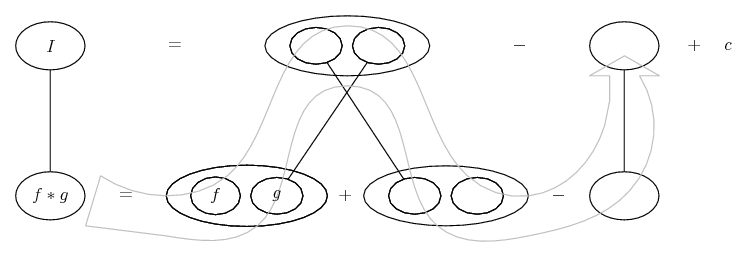# Thread: 2011 MIT Integration Bee Qualifying Test

1. haha, i saw that video once, it's a fairly easy integral, so i consider they are trying to prove your intelligence and speed altogether.

2.Originally Posted by TheCoffeeMachineIt took the winner about 30 seconds to do $\displaystyle \int \frac{x^4}{1-x^2}\;{dx}$. What do you think?

Some books (for example Demidovich) include the following formula in the Table for immediate integrals:

$\displaystyle \displaystyle\int \dfrac{dx}{a^2-x^2}=\dfrac{1}{2a}\ln \left |{\dfrac{a+x}{a-x}}\right |+C\quad (a\neq 0)$

In that case, and taking into account that we needn't an elegant development, and that the euclidean division is almost immediate, the integral can be computed in less than 20 seconds.Fernando Revilla

3. Nice case of the 'addition/subtraction' method in no. 14.

$\displaystyle \displaystyle{\ \ \frac{1}{x^2(x^4 + 1)^{3/4}}}$

$\displaystyle \displaystyle{=\ \frac{(x^4 + 1) - x^4}{x^2(x^4 + 1)^{3/4}}$

$\displaystyle \displaystyle{=\ \frac{(x^4 + 1)^{3/4}(x^4 + 1)^{1/4} - x^4}{x^2(x^4 + 1)^{3/4}}$

$\displaystyle \displaystyle{=\ \frac{(x^4 + 1)^{1/4}}{x^2}\ -\ \frac{x^2}{(x^4 + 1)^{3/4}}$

As with no.s 2 and 16, start parts with one half of it and it's all over 'too' soon...... where (key in spoiler) ...

Spoiler:... is the product rule. Straight continuous lines differentiate downwards (integrate up) with respect to x. And,... is lazy integration by parts, doing without u and v.

_________________________________________

Don't integrate - balloontegrate!

Balloon Calculus; standard integrals, derivatives and methods

Balloon Calculus Drawing with LaTeX and Asymptote!

4. Is this party over? Oh well, anyway... no.11... where (key in spoiler) ...

Spoiler:... is the product rule. Straight continuous lines differentiate downwards (integrate up) with respect to x. And,... is lazy integration by parts, doing without u and v.

_________________________________________

Don't integrate - balloontegrate!

Balloon Calculus; standard integrals, derivatives and methods

Balloon Calculus Drawing with LaTeX and Asymptote!

5. 2) $\displaystyle \displaystyle \int 2 \ln x + \ln^2 x\, dx = \int \left( x \ln^2x \right)'\,dx$

6. $\displaystyle \int \sin[(n+1)x] \sin^{n-1}x {dx} = \int [\sin(nx)\cos x+\cos(nx)\sin x ] \sin^{n-1}x {dx}$
$\displaystyle = \frac{1}{n} \int \sin(nx) d\sin^n x+\int \cos(nx) \sin^n x {dx} =\frac{1}{n} \sin(nx)\sin^n x + c$

7. I thought the following three integrals were very similar to those in the test:

1. $\displaystyle \int\frac{2x\cos{x}+\sin{x}}{4\sqrt{x}\sqrt{\sin{x }\sqrt{x}}}\;{dx}.$

2. $\displaystyle \int \frac{2\sin^{-1}{x}+2\cos^{-1}{x}}{\sqrt{1-x^2}}\;{dx}.$

3. $\displaystyle \int\frac{1}{x+x\log^2{x}}\;{dx}$.

All three can be solved without explicit substitution (the last being the easiest).

8.Originally Posted by TheCoffeeMachine2. $\displaystyle \int \frac{2\sin^{-1}{x}+2\cos^{-1}{x}}{\sqrt{1-x^2}}\;{dx}.$
Is this an accident? It equals $\displaystyle \displaystyle \pi\int\frac{dx}{\sqrt{1-x^2}}=\pi\arcsin(x)+C$

9.Originally Posted by Drexel28Is this an accident? It equals $\displaystyle \displaystyle \pi\int\frac{dx}{\sqrt{1-x^2}}=\pi\arcsin(x)+C$
Doh, I didn't notice that!I was thinking more along the lines of:

\displaystyle \begin{aligned} \displaystyle \int \frac{2\sin^{-1}{x}+2\cos^{-1}{x}}{\sqrt{1-x^2}}\;{dx} & = \int \frac{2\sin^{-1}{x}}{\sqrt{1-x^2}}+\frac{2\cos^{-1}{x}}{\sqrt{1-x^2}}\;{dx} \\& = \int 2\sin^{-1}{x}(\sin^{-1}{x})' -2\cos^{-1}{x}(\cos^{-1}{x})'\;{dx} \\& = \int \left[\left(\sin^{-1}{x}\right)^2\right]'-\left[\left(\cos^{-1}{x}\right)^2\right]' \;{dx} \\& = \left(\sin^{-1}{x}\right)^2-\left(\cos^{-1}{x}\right)^2+k. \end{aligned}

,
,

,

,

,

# MIT integration problems

Click on a term to search for related topics.

#### Search Tags

2011, bee, integration, mit, qualifying, test